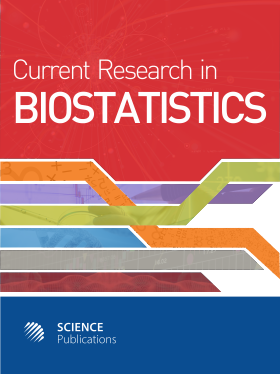Frequency: Continuous
ISSN: 2524-2210 (Print)
ISSN: 2524-2229 (Online)
Research Article Open Access

# Measures of Explained Variation and the Base-Rate Problem for Logistic Regression

Dinesh Sharma1, Dan McGee2 and B.M. Golam Kibria3
• 1 James Madison University, United States
• 2 Florida State University, United States
• 3 Florida International University, United States

## Abstract

Problem statement: Logistic regression, perhaps the most frequently used regression model after the General Linear Model (GLM), is extensively used in the field of medical science to analyze prognostic factors in studies of dichotomous outcomes. Unlike the GLM, many different proposals have been made to measure the explained variation in logistic regression analysis. One of the limitations of these measures is their dependency on the incidence of the event of interest in the population. This has clear disadvantage, especially when one seeks to compare the predictive ability of a set of prognostic factors in two subgroups of a population. Approach: The purpose of this article is to study the base-rate sensitivity of several R2 measures that have been proposed for use in logistic regression. We compared the base-rate sensitivity of thirteen R2 type parametric and nonparametric statistics. Since a theoretical comparison was not possible, a simulation study was conducted for this purpose. We used results from an existing dataset to simulate populations with different base-rates. Logistic models are generated using the covariate values from the dataset. Results: We found nonparametric R2 measures to be less sensitive to the base-rate as compared to their parametric counterpart. Logistic regression is a parametric tool and use of the nonparametric R2 may result inconsistent results. Among the parametric R2 measures, the likelihood ratio R2 appears to be least dependent on the base-rate and has relatively superior interpretability as a measure of explained variation. Conclusion/Recommendations: Some potential measures of explained variation are identified which tolerate fluctuations in base-rate reasonably well and at the same time provide a good estimate of the explained variation on an underlying continuous variable. It would be, however, misleading to draw strong conclusions based only on the conclusions of this research only.

Current Research in Biostatistics
Volume 2 No. 1, 2011, 11-19

DOI:

Submitted On: 31 January 2012 Published On: 25 February 2012

How to Cite: Sharma, D., McGee, D. & Kibria, B. G. (2011). Measures of Explained Variation and the Base-Rate Problem for Logistic Regression. Current Research in Biostatistics, 2(1), 11-19. https://doi.org/10.3844/amjbsp.2011.11.19

• 2,109 Views